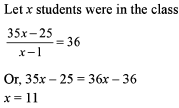Courses

# Test: Average- 3

## 15 Questions MCQ Test General Aptitude for GATE 2020 | Test: Average- 3

Description
This mock test of Test: Average- 3 for Quant helps you for every Quant entrance exam. This contains 15 Multiple Choice Questions for Quant Test: Average- 3 (mcq) to study with solutions a complete question bank. The solved questions answers in this Test: Average- 3 quiz give you a good mix of easy questions and tough questions. Quant students definitely take this Test: Average- 3 exercise for a better result in the exam. You can find other Test: Average- 3 extra questions, long questions & short questions for Quant on EduRev as well by searching above.
QUESTION: 1

### Find the average age of a family of five members, whose ages are 42, 49, 56, 63 and 35 years respectively.

Solution: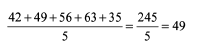QUESTION: 2

### The average of five numbers is 39.20 and the average of three of these numbers is 41. Find the average of the remaining two numbers.

Solution: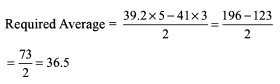QUESTION: 3

### What is the average of the first 15 multiples of 3?

Solution:

The first 15 multiples of 3 will be

3, 6, 9, 12, 15, 18, 21, 24, 27, 30, 33, 36, 39, 42, 45

Average = (Sum of multiples)/(Total number of multiples)

Sum of multiples = 360

∴ Average = 360/15 = 24

QUESTION: 4

The average of seven consecutive even numbers is 10. If the next three even numbers are included, then what is the new average?

Solution: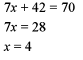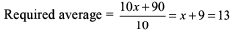QUESTION: 5

In a test, the average marks of five students was found to be 50. However during cross checking of the papers, it was discovered that the mark of one student was misread as 84 instead of 48. Find the correct average.

Solution: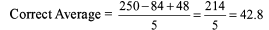QUESTION: 6

In a prep school, the average weight o f 30 girls in a class of 50 students is 16 kg and that of the remaining students is 15.5 kg. What is the average weight of the all the students in the class?

Solution: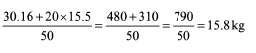QUESTION: 7

In what ratio must 35% spirit solution be mixed with pure spirit to get a resultant solution of 56% spirit?

Solution: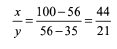QUESTION: 8

Three litres of pure milk is added to 10 litres of a milk solution containing 9% milk. Find the concentration of the resultant solution.

Solution: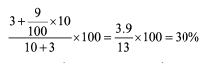QUESTION: 9

Parul Jaiswal has Rs 11,200 in Rs 100 and Rs 500 denomination. What is the ratio of the number of notes, of the two types, if she has a total of 40 notes with her?

Solution: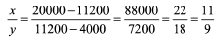QUESTION: 10

A shrewd milkman mixes water and milk in the ratio of 2:3. What part of this mixture should be removed and replaced with water so that the solution contains water and milk in the ratio 1:1?

Solution:

Assume  5L mix

=> 2L water + 3 L milk

XL  mixture is removed.

2x/ 5 water  +  3x / 5 remaining

( 2- 2x / 5 + x)  water  +  ( 3- 3x /5 ) milk

New ratio, 1:1

( 2- 2x / 5 + x) =  ( 3- 3x / 5)

=> 10-2x +5x = 15-3x

=>  6x = 5

=>  x =  5 / 6L

In 5L ,    5 / 6 is removed

1 L  =>   1 / 6 is removed.

QUESTION: 11

Neo is going to NDLS from PNBE by his car at a speed of 40 km/h. While coming back, he returns with a speed of x km/h. What should be the value of x so that his average speed during the intre journey is 80 km/h?

Solution: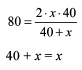Hence, not possible Alternatively, the average speed for the whole journey has to be less than double the speed of one- side. In this case, speed in one direction = 40 kmph, hence average speed for the whole journey has to be less than 80 kmph.

QUESTION: 12

I went to a hotel along with 12 friends. I paid Rs 145 and all the others paid an equal amount. In the end, we did some calculations and found that the average sum paid by all of us was Rs 5 more than what was originally paid by each of my friends. How much money did each of my friends pay?

Solution:

(140+12x)/13 = x + 5
145 + 12x = 13x + 65
x = 80

QUESTION: 13

Mr Anant Roy, the renowned author, recently got his new novel released. To his utter dismay he found that for the 1,007 pages on an average there were 2 mistakes every page. While, in the first 612 pages there were only 434 mistakes, they seemed to increase for the latter pages. Find the average number of mistakes per page for the remaining pages.

Solution:

Total mistakes = 1007 x 2 = 2014

Let x be average mistake per page for the remaining pages

434 + 395x = 2014

395x = 1580 x = 4

QUESTION: 14

The average score of a cricketer in a certain number of innings is 44. He then played another eight innings in which he got out at 97, 3, 23, 0, 68, 40, 50 and 71 runs respectively. Then, which of the following statements is true regarding his new average score in all the innings together?

Solution:

Avg of 8 new innings : (97 + 3 + 23 + 0 + 68 + 40 + 50 + 71)/8
= 352/8
= 44
Therefore, old inning is same as new inning.

QUESTION: 15

Read the passage befow and solve the questions based on it.
The average age of the students in a class is 35 years

Q.

If a student whose age was 25 is absent from the class, the average of those present goes up by 1. How many students were there in the class originally?

Solution: